# 2d地图编辑器 unity3d

## 【跟我一起学Unity3D】做一个2D的90坦克大战之地图编辑器

2014-10-31 21:20:10 baijiajie2012 阅读数 4072

```1.地图编辑器
2.道具系统
3.简单AI系统
4.计分器```

------------------------------------------------------------------------------------------------------------------------------------------

``` <item>
<name>gras</name>
<position_x>-1.2</position_x>
<position_y>-3</position_y>
</item>```

```<data>
<items>
<item>
<name>gras</name>
<position_x>-1.2</position_x>
<position_y>-3</position_y>
</item>
</items>
</data>```

```    bool SaveInXml()
{
if (File.Exists(m_sXmlPath))
{
File.Delete(m_sXmlPath);
}
XmlDocument xmlDoc = new XmlDocument();
XmlElement data = xmlDoc.CreateElement("data");
xmlDoc.AppendChild(data);
//地图数据
XmlElement xml_items = xmlDoc.CreateElement("items");
data.AppendChild(xml_items);
foreach (GameObject item in m_Items)
{
Debug.Log("要保存的数据：" + item.name + "位置：" + item.transform.position.x + "," + item.transform.position.y);

//save in xml file
XmlElement xml_item = xmlDoc.CreateElement("item");
xml_items.AppendChild(xml_item);
XmlElement name = xmlDoc.CreateElement("name");
name.InnerText = item.name;
xml_item.AppendChild(name);

XmlElement position_x = xmlDoc.CreateElement("position_x");
position_x.InnerText = item.transform.position.x.ToString();
xml_item.AppendChild(position_x);

XmlElement position_y = xmlDoc.CreateElement("position_y");
position_y.InnerText = item.transform.position.y.ToString();
xml_item.AppendChild(position_y);
}
xmlDoc.Save(m_sXmlPath);
Debug.Log("创建XML完毕");
m_Items.Clear();
return true;
}```

```    void ReadMap(XmlNode xml_items)
{
XmlNodeList items = xml_items.SelectNodes("item");
foreach (XmlNode current_node in items)
{
XmlNode current_name = current_node.SelectSingleNode("name");
XmlNode position_x = current_node.SelectSingleNode("position_x");
XmlNode position_y = current_node.SelectSingleNode("position_y");

Vector3 psition = new Vector3(float.Parse(position_x.InnerText), float.Parse(position_y.InnerText), -1);

if (current_name.InnerText == "wall")
{
m_readItem = Instantiate(m_ItemWall, psition, Quaternion.identity) as GameObject;
}

else if (current_name.InnerText == "gras")
{
m_readItem = Instantiate(m_ItemGras, psition, Quaternion.identity) as GameObject;
}

else if (current_name.InnerText == "steel")
{
m_readItem = Instantiate(m_ItemSteel, psition, Quaternion.identity) as GameObject;
}

else if (current_name.InnerText == "water")
{
m_readItem = Instantiate(m_ItemWater, psition, Quaternion.identity) as GameObject;
}

else continue;

}
}```

```    void Update()
{
//检测鼠标左键的拾取
if (Input.GetMouseButtonDown(0))
{
//鼠标的屏幕坐标空间位置转射线
m_ray = Camera.main.ScreenPointToRay(Input.mousePosition);
m_rayhit = Physics2D.GetRayIntersection(m_ray);
//射线检测，相关检测信息保存到RaycastHit 结构中
if (m_rayhit)
{
//打印射线碰撞到的对象的名称
Debug.Log(m_rayhit.collider.gameObject.name);
if (m_rayhit.collider.gameObject.name == "walls" ||
m_rayhit.collider.gameObject.name == "grass" ||
m_rayhit.collider.gameObject.name == "steels" ||
m_rayhit.collider.gameObject.name == "waters")
{
m_clickItem = Instantiate(m_rayhit.collider.gameObject, m_rayhit.collider.transform.position, Quaternion.identity) as GameObject;
m_clickItem.name = m_rayhit.collider.gameObject.name.Substring(0, m_rayhit.collider.gameObject.name.Length - 1);
//m_clickItem.tag = m_clickItem.name; //ERROR: not define tag in editor
}
else if (m_rayhit.collider.gameObject.name == "wall" ||
m_rayhit.collider.gameObject.name == "gras" ||
m_rayhit.collider.gameObject.name == "steel" ||
m_rayhit.collider.gameObject.name == "water")
{
m_clickItem = m_rayhit.collider.gameObject;
}
else
{
m_clickItem = null;
}
}
else
{
m_clickItem = null;
}
}
if (Input.GetMouseButton(0))
{
if (m_clickItem != null)
{
m_clickItem.transform.position = SetPointInMap(new Vector3(camera.ScreenToWorldPoint(Input.mousePosition).x, camera.ScreenToWorldPoint(Input.mousePosition).y, -1));
}
}
if (Input.GetMouseButtonUp(0))
{
if (m_clickItem != null)
{
Vector3 cur_point = SetPointInMap(new Vector3(camera.ScreenToWorldPoint(Input.mousePosition).x, camera.ScreenToWorldPoint(Input.mousePosition).y, -1));
if (m_Map.collider2D.OverlapPoint(cur_point))
{
m_clickItem.transform.position = cur_point;
}
else	//在地图外就销毁掉
{
Destroy(m_clickItem);
}
}
}
}```

2d地图编辑器 unity3d 相关内容

## Unity~Tiled地图编辑器和Tiled2Unity 插件导入地图的简单使用

2018-08-28 15:35:46 jinxiul5 阅读数 4792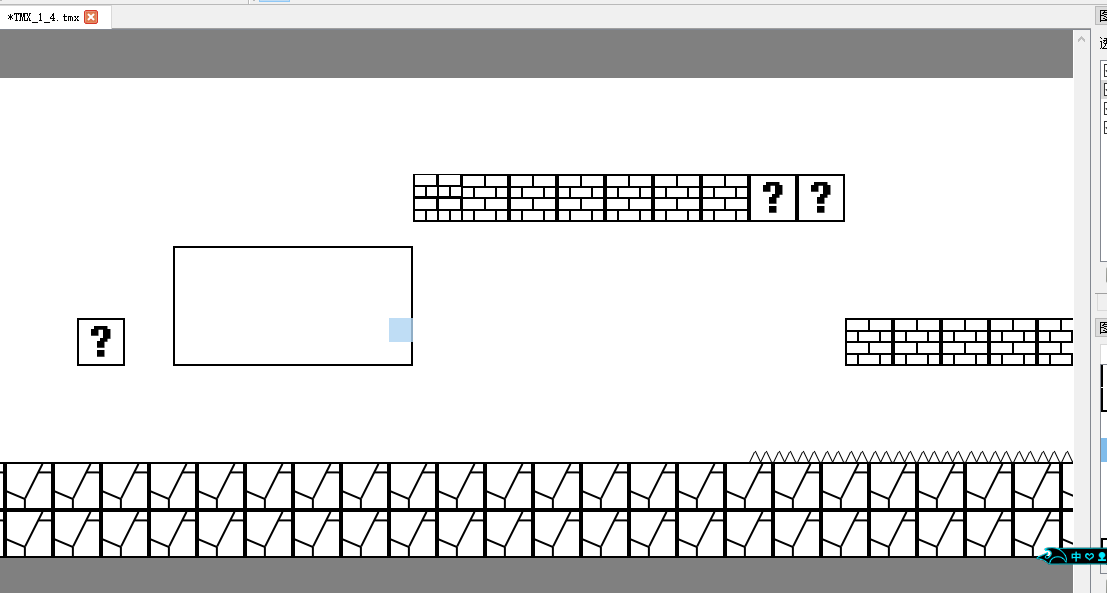## Tilde2Unity的使用 :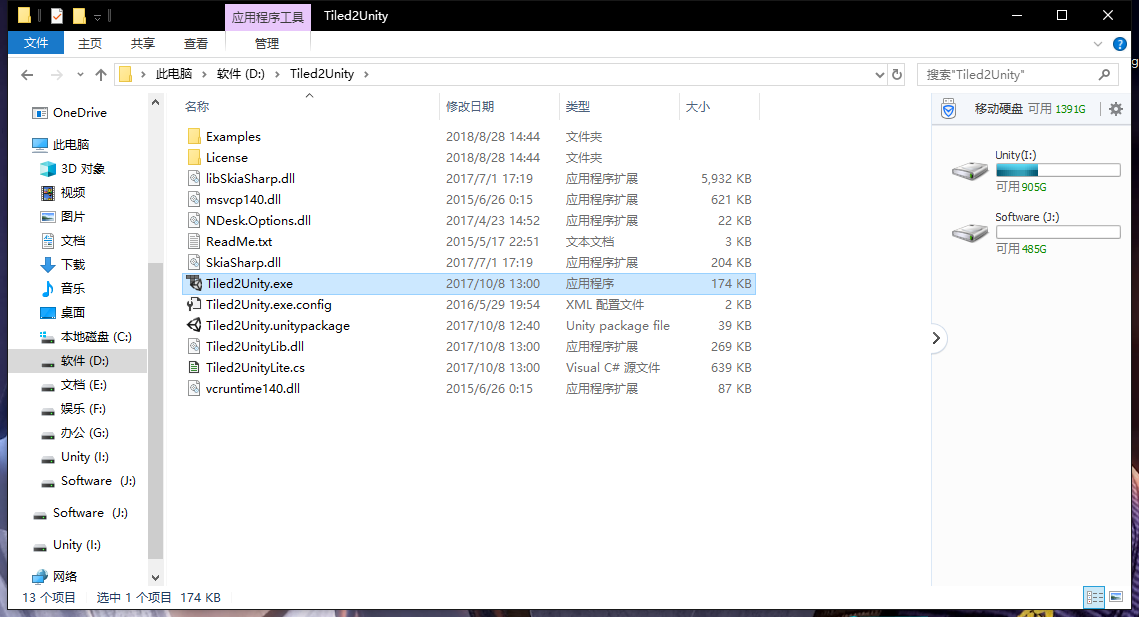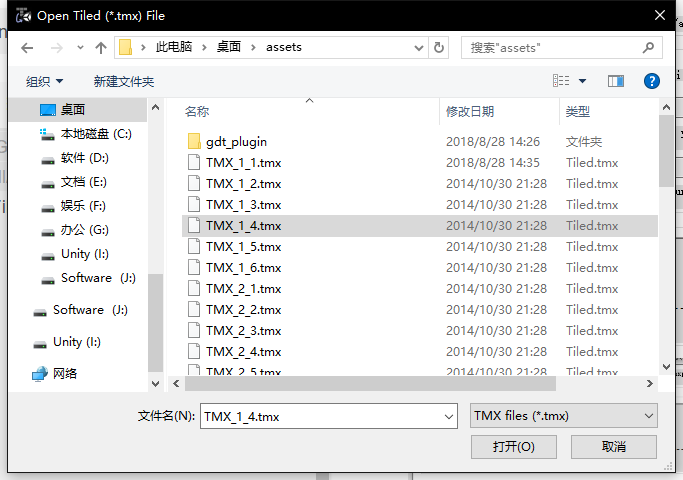单击打开就Ok了

Help–> Import Unity Package to Project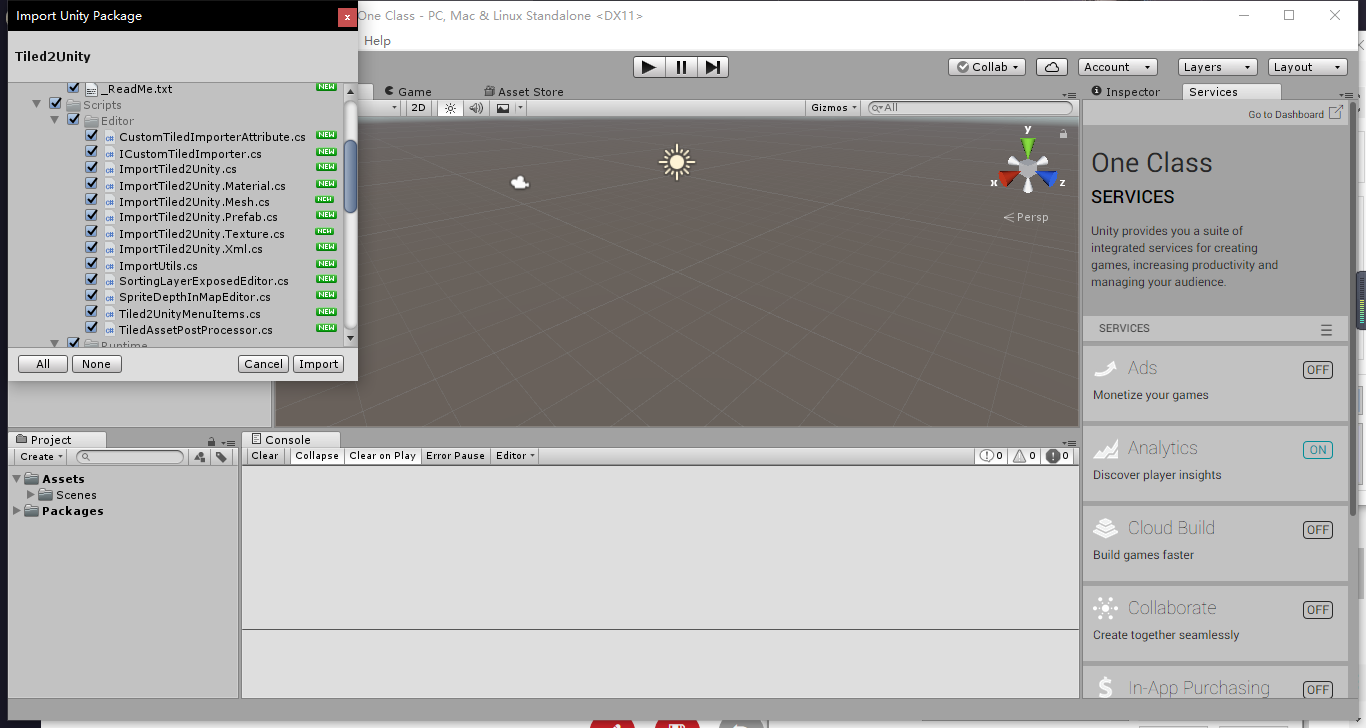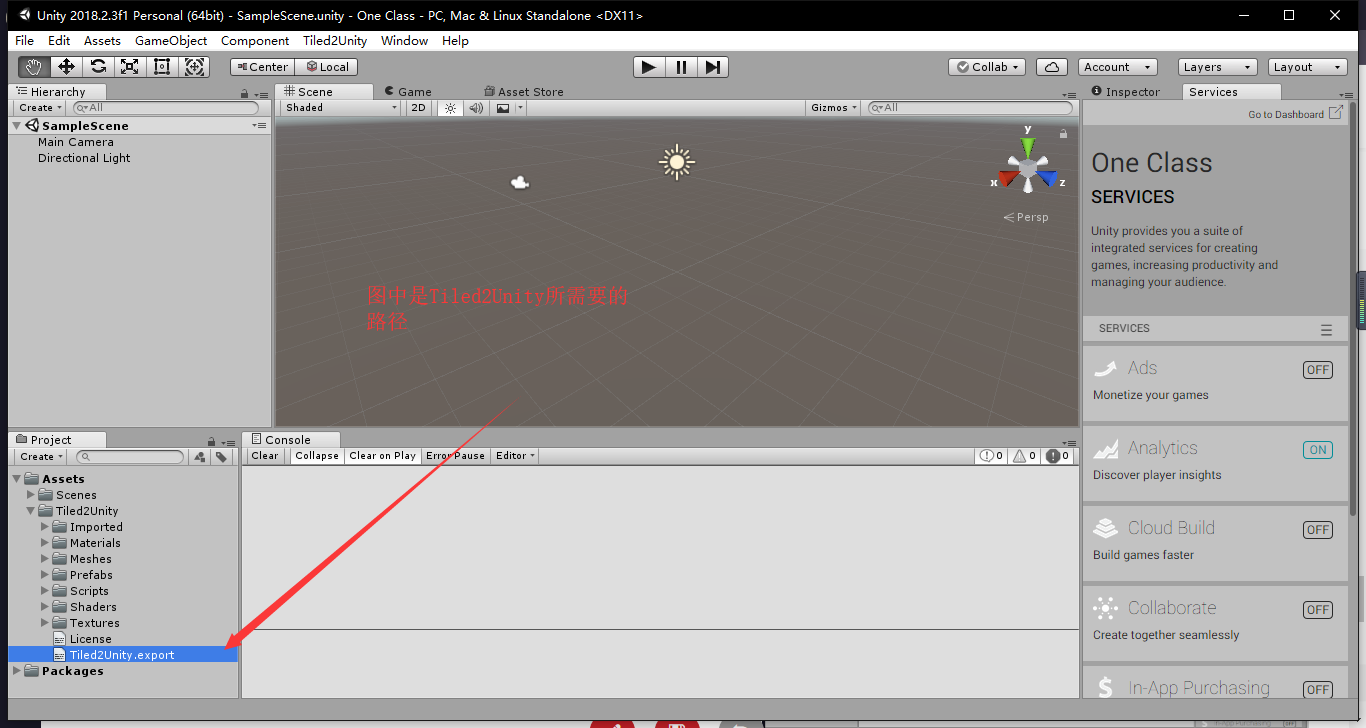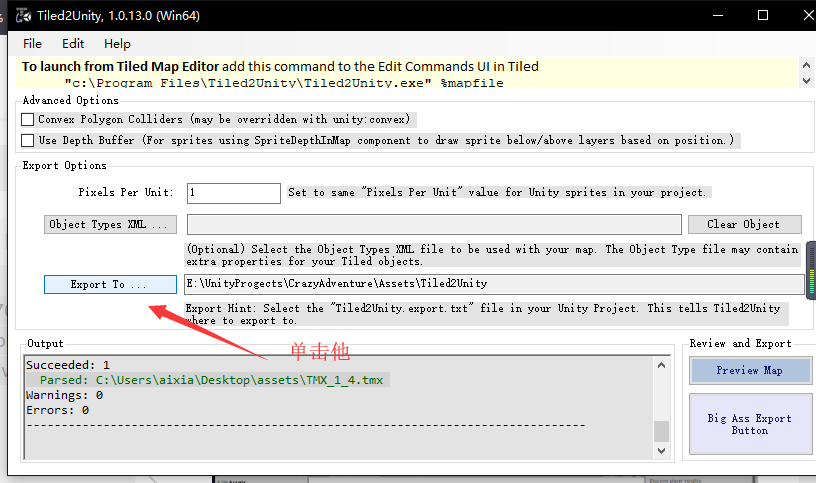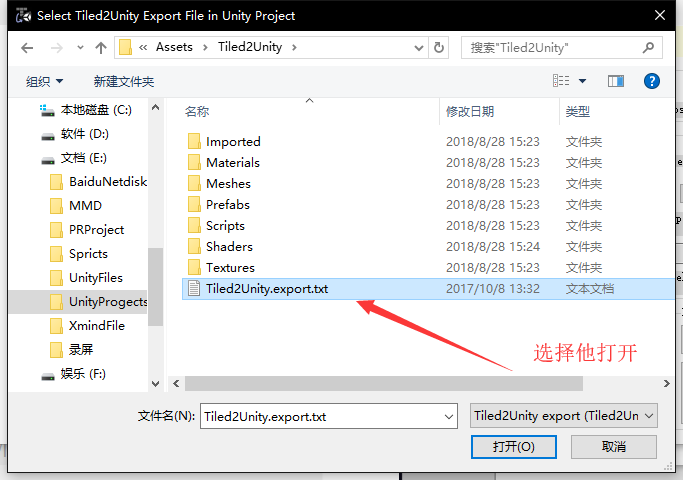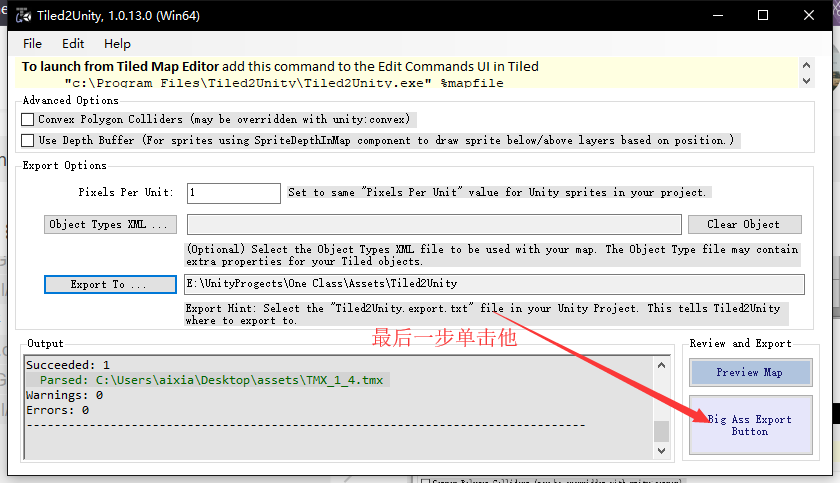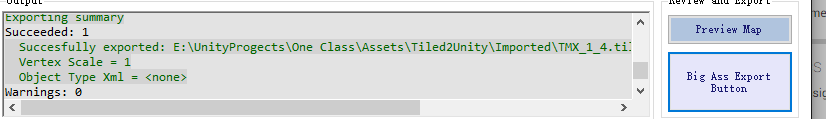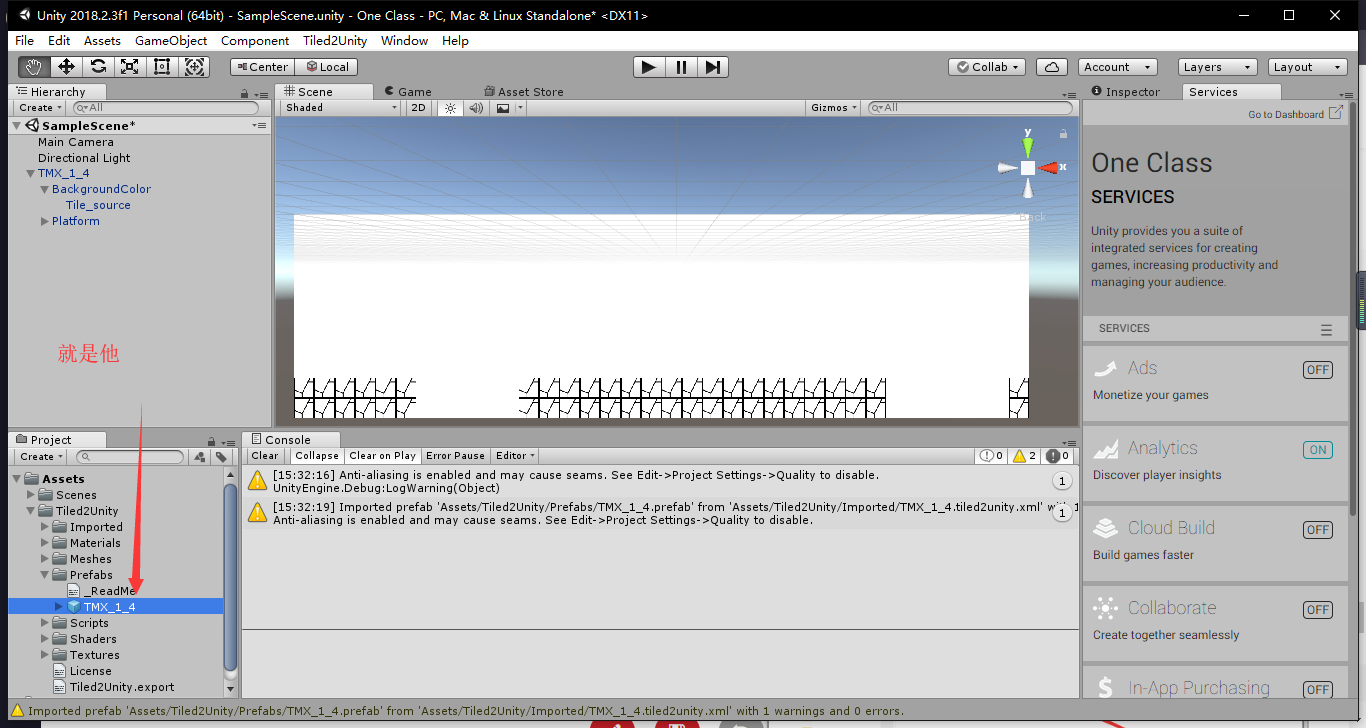2d地图编辑器 unity3d 相关内容

## Unity3D实现地图编辑器的插件

2015-10-17 08:35:43 u011611902 阅读数 16278

nway 电话面试总的来说很糟糕，有些事没沟通清楚，加上手机出了问题。很慌的。2个资源岛，地表信息一样的，但建筑位置不一样的。

Editor，unity=>Help=>ScriptReference=>搜索框里输入Editor；

2d地图编辑器 unity3d 相关内容

## Unity3d开发——保卫萝卜学习笔记一地图编辑器开发

2017-05-20 15:47:29 likanglk521 阅读数 6087
``````   一直在学习，几乎边学边忘，再也不想打王者农药了，现在打卡学习持续写博客，现在在开发学习保卫萝卜，这篇文章关于地图编辑器的开发，在项目中不能是无限个场景，用读取xml文件的方法可以说是能实现无限关卡，同时节省很多开发时间缩短周期
``````

## 一.目录结构## 二.创建数据类(放置在Data目录)

1. 首先看一下XML文件内容2. 根据xml文件节点创建一些数据类，首先创建Point.cs脚本,放置在Data目录中

``````using UnityEngine;
using System.Collections;

//格子坐标
public class Point
{
public int X;
public int Y;

public Point(int x, int y)
{
this.X = x;
this.Y = y;
}
}``````

3.创建Tile.cs脚本

``````using System;
using System.Collections.Generic;
using System.Linq;
using System.Text;

//格子信息
public class Tile
{
public int X;
public int Y;
public bool CanHold; //是否可以放置塔
public object Data; //格子所保存的数据

public Tile(int x, int y)
{
this.X = x;
this.Y = y;
}

public override string ToString()
{
return string.Format("[X:{0},Y:{1},CanHold:{2}]",
this.X,
this.Y,
this.CanHold
);
}
}
``````

4.创建Round.cs脚本

``````using System;
using System.Collections.Generic;
using System.Linq;
using System.Text;

public class Round
{
public int Monster; //怪物类型ID
public int Count;   //怪物数量

public Round(int monster, int count)
{
this.Monster = monster;
this.Count = count;
}
}``````

5.根据xml文件信息创建关卡类Level.cs

``````using UnityEngine;
using System.Collections;
using System.Collections.Generic;

public class Level
{
//名字
public string Name;

//背景
public string Background;

//路径

//金币
public int InitScore;

//炮塔可放置的位置
public List<Point> Holder = new List<Point>();

//怪物行走的路径
public List<Point> Path = new List<Point>();

//出怪回合信息
public List<Round> Rounds = new List<Round>();
}``````

## 三.创建读写XML工具类

``````using UnityEngine;
using System.IO;
using System.Collections;
using System.Collections.Generic;
using System.Text;
using System.Xml;

public class Tools
{
//读取关卡列表
public static List<FileInfo> GetLevelFiles()
{
string[] files = Directory.GetFiles(Consts.LevelDir, "*.xml");

List<FileInfo> list = new List<FileInfo>();
for (int i = 0; i < files.Length; i++)
{
FileInfo file = new FileInfo(files[i]);
}
return list;
}

//填充Level类数据
public static void FillLevel(string fileName, ref Level level)
{
FileInfo file = new FileInfo(fileName);

XmlDocument doc = new XmlDocument();

level.Name = doc.SelectSingleNode("/Level/Name").InnerText;
level.Background = doc.SelectSingleNode("/Level/Background").InnerText;
level.InitScore = int.Parse(doc.SelectSingleNode("/Level/InitScore").InnerText);

XmlNodeList nodes;

nodes = doc.SelectNodes("/Level/Holder/Point");
for (int i = 0; i < nodes.Count; i++)
{
XmlNode node = nodes[i];
Point p = new Point(
int.Parse(node.Attributes["X"].Value),
int.Parse(node.Attributes["Y"].Value));

}

nodes = doc.SelectNodes("/Level/Path/Point");
for (int i = 0; i < nodes.Count; i++)
{
XmlNode node = nodes[i];

Point p = new Point(
int.Parse(node.Attributes["X"].Value),
int.Parse(node.Attributes["Y"].Value));

}

nodes = doc.SelectNodes("/Level/Rounds/Round");
for (int i = 0; i < nodes.Count; i++)
{
XmlNode node = nodes[i];

Round r = new Round(
int.Parse(node.Attributes["Monster"].Value),
int.Parse(node.Attributes["Count"].Value)
);

}

sr.Close();
sr.Dispose();
}

//保存关卡
public static void SaveLevel(string fileName, Level level)
{
StringBuilder sb = new StringBuilder();
sb.AppendLine("<?xml version=\"1.0\" encoding=\"utf-8\"?>");
sb.AppendLine("<Level>");

sb.AppendLine(string.Format("<Name>{0}</Name>", level.Name));
sb.AppendLine(string.Format("<Background>{0}</Background>", level.Background));
sb.AppendLine(string.Format("<InitScore>{0}</InitScore>", level.InitScore));

sb.AppendLine("<Holder>");
for (int i = 0; i < level.Holder.Count; i++)
{
sb.AppendLine(string.Format("<Point X=\"{0}\" Y=\"{1}\"/>", level.Holder[i].X, level.Holder[i].Y));
}
sb.AppendLine("</Holder>");

sb.AppendLine("<Path>");
for (int i = 0; i < level.Path.Count; i++)
{
sb.AppendLine(string.Format("<Point X=\"{0}\" Y=\"{1}\"/>", level.Path[i].X, level.Path[i].Y));
}
sb.AppendLine("</Path>");

sb.AppendLine("<Rounds>");
for (int i = 0; i < level.Rounds.Count; i++)
{
sb.AppendLine(string.Format("<Round Monster=\"{0}\" Count=\"{1}\"/>", level.Rounds[i].Monster, level.Rounds[i].Count));
}
sb.AppendLine("</Rounds>");

sb.AppendLine("</Level>");

string content = sb.ToString();

StreamWriter sw = new StreamWriter(fileName, false, Encoding.UTF8);
sw.Write(content);
sw.Flush();
sw.Dispose();
}

//加载图片
public static IEnumerator LoadImage(string url, SpriteRenderer render)
{
WWW www = new WWW(url);

while (!www.isDone)
yield return www;

Texture2D texture = www.texture;
Sprite sp = Sprite.Create(
texture,
new Rect(0, 0, texture.width, texture.height),
new Vector2(0.5f, 0.5f));
render.sprite = sp;
}
}
``````

## 三.创建编辑器Editor类

1.创建常量类Consts.cs

``````using System;
using System.Collections.Generic;
using System.Linq;
using System.Text;
using UnityEngine;

public static class Consts
{
//目录
public static readonly string LevelDir = Application.dataPath + @"\Game\Res\Levels";
public static readonly string MapDir = Application.dataPath + @"\Game\Res\Maps";
}``````

2.创建Map.cs,此脚本挂在层级试图hierarchy中的Map上

``````using UnityEngine;
using System;
using System.Collections;
using System.Collections.Generic;

public class Map : MonoBehaviour
{
#region 常量
public const int RowCount = 8;   //行数
public const int ColumnCount = 12; //列数
#endregion

#region 字段
float MapWidth;//地图宽
float MapHeight;//地图高

float TileWidth;//格子宽
float TileHeight;//格子高

List<Tile> m_grid = new List<Tile>(); //格子集合
List<Tile> m_road = new List<Tile>(); //路径集合

Level m_level; //关卡数据

public bool DrawGizmos = true; //是否绘制网格
#endregion

#region 属性

public Level Level
{
get { return m_level; }
}

public string BackgroundImage
{
set
{
SpriteRenderer render = transform.Find("Background").GetComponent<SpriteRenderer>();
}
}

{
set
{
}
}

public List<Tile> Grid
{
get { return m_grid; }
}

{
}

//怪物的寻路路径
public Vector3[] Path
{
get
{
List<Vector3> m_path = new List<Vector3>();
for (int i = 0; i < m_road.Count; i++)
{
Vector3 point = GetPosition(t);
}
return m_path.ToArray();
}
}

#endregion

#region 方法
{
//清除当前状态
Clear();

//保存
this.m_level = level;

//加载图片
this.BackgroundImage = "file://" + Consts.MapDir + "/" + level.Background;

//寻路点
for (int i = 0; i < level.Path.Count; i++)
{
Point p = level.Path[i];
Tile t = GetTile(p.X, p.Y);
}

//炮塔点
for (int i = 0; i < level.Holder.Count; i++)
{
Point p = level.Holder[i];
Tile t = GetTile(p.X, p.Y);
t.CanHold = true;
}
}

//清除塔位信息
public void ClearHolder()
{
foreach (Tile t in m_grid)
{
if(t.CanHold)
t.CanHold = false;
}
}

//清除寻路格子集合
{
}

//清除所有信息
public void Clear()
{
m_level = null;
ClearHolder();
}

#endregion

#region Unity回调
//只在运行期起作用
void Awake()
{
//计算地图和格子大小
CalculateSize();

//创建所有的格子
for (int i = 0; i < RowCount; i++)
for (int j = 0; j < ColumnCount; j++)
}

//只在编辑器里起作用
void OnDrawGizmos()
{
if (!DrawGizmos)
return;

//计算地图和格子大小
CalculateSize();

//绘制格子
Gizmos.color = Color.green;

//绘制行
for (int row = 0; row <= RowCount; row++)
{
Vector2 from = new Vector2(-MapWidth / 2, -MapHeight / 2 + row * TileHeight);
Vector2 to = new Vector2(-MapWidth / 2 + MapWidth, -MapHeight / 2 + row * TileHeight);
Gizmos.DrawLine(from, to);
}

//绘制列
for (int col = 0; col <= ColumnCount; col++)
{
Vector2 from = new Vector2(-MapWidth / 2 + col * TileWidth, MapHeight / 2);
Vector2 to = new Vector2(-MapWidth / 2 + col * TileWidth, -MapHeight / 2);
Gizmos.DrawLine(from, to);
}

foreach (Tile t in m_grid)
{
if (t.CanHold)
{
Vector3 pos = GetPosition(t);
Gizmos.DrawIcon(pos, "holder.png", true);
}
}

Gizmos.color = Color.red;
for (int i = 0; i < m_road.Count; i++)
{
//起点
if (i == 0)
{
}

//终点
{
}

//红色的连线
if (m_road.Count > 1 && i != 0)
{
Vector3 from = GetPosition(m_road[i - 1]);
Gizmos.DrawLine(from, to);
}
}
}
#endregion

#region 帮助方法
//计算地图大小，格子大小
void CalculateSize()
{
Vector3 leftDown = new Vector3(0, 0);
Vector3 rightUp = new Vector3(1, 1);

Vector3 p1 = Camera.main.ViewportToWorldPoint(leftDown);
Vector3 p2 = Camera.main.ViewportToWorldPoint(rightUp);

MapWidth = (p2.x - p1.x);
MapHeight = (p2.y - p1.y);

TileWidth = MapWidth / ColumnCount;
TileHeight = MapHeight / RowCount;
}

//获取格子中心点所在的世界坐标
Vector3 GetPosition(Tile t)
{
return new Vector3(
-MapWidth / 2 + (t.X + 0.5f) * TileWidth,
-MapHeight / 2 + (t.Y + 0.5f) * TileHeight,
0
);
}

//根据格子索引号获得格子
Tile GetTile(int tileX, int tileY)
{
int index = tileX + tileY * ColumnCount;

if (index < 0 || index >= m_grid.Count)
return null;

return m_grid[index];
}

//获取鼠标下面的格子
Tile GetTileUnderMouse()
{
Vector2 wordPos = GetWorldPosition();
int col = (int)((wordPos.x + MapWidth / 2) / TileWidth);
int row = (int)((wordPos.y + MapHeight / 2) / TileHeight);
return GetTile(col, row);
}

//获取鼠标所在位置的世界坐标
Vector3 GetWorldPosition()
{
Vector3 viewPos = Camera.main.ScreenToViewportPoint(Input.mousePosition);
Vector3 worldPos = Camera.main.ViewportToWorldPoint(viewPos);
return worldPos;
}
#endregion
}``````

3.在Editor文件夹下创建编辑器类MapEditor.cs

``````using UnityEngine;
using UnityEditor;
using System.IO;
using System.Collections;
using System.Collections.Generic;

[CustomEditor(typeof(Map))]
public class MapEditor : Editor
{
[HideInInspector]
public Map Map = null;

//关卡列表
List<FileInfo> m_files = new List<FileInfo>();

//当前编辑的关卡索引号
int m_selectIndex = -1;

public override void OnInspectorGUI()
{
base.OnInspectorGUI();

if(Application.isPlaying)
{
//关联的Mono脚本组件
Map = target as Map;

EditorGUILayout.BeginHorizontal();
int currentIndex = EditorGUILayout.Popup(m_selectIndex, GetNames(m_files));
if (currentIndex != m_selectIndex)
{
m_selectIndex = currentIndex;

//加载关卡
}
if (GUILayout.Button("读取列表"))
{
//读取关卡列表
}
EditorGUILayout.EndHorizontal();

EditorGUILayout.BeginHorizontal();
if (GUILayout.Button("清除塔点"))
{
Map.ClearHolder();
}
if (GUILayout.Button("清除路径"))
{
}
EditorGUILayout.EndHorizontal();

if (GUILayout.Button("保存数据"))
{
//保存关卡
SaveLevel();
}
}

if (GUI.changed)
EditorUtility.SetDirty(target);
}

{
//清除状态
Clear();

//加载列表
m_files = Tools.GetLevelFiles();

//默认加载第一个关卡
if (m_files.Count > 0)
{
m_selectIndex = 0;
}
}

{
FileInfo file = m_files[m_selectIndex];

Level level = new Level();
Tools.FillLevel(file.FullName, ref level);

}

void SaveLevel()
{
//获取当前加载的关卡
Level level = Map.Level;

//临时索引点
List<Point> list = null;

//收集放塔点
list = new List<Point>();
for (int i = 0; i < Map.Grid.Count; i++)
{
Tile t = Map.Grid[i];
if (t.CanHold)
{
Point p = new Point(t.X, t.Y);
}
}
level.Holder = list;

//收集寻路点
list = new List<Point>();
for (int i = 0; i < Map.Road.Count; i++)
{
Point p = new Point(t.X, t.Y);
}
level.Path = list;

//路径
string fileName = m_files[m_selectIndex].FullName;

//保存关卡
Tools.SaveLevel(fileName, level);

//弹框提示
EditorUtility.DisplayDialog("保存关卡数据", "保存成功", "确定");
}

void Clear()
{
m_files.Clear();
m_selectIndex = -1;
}

string[] GetNames(List<FileInfo> files)
{
List<string> names = new List<string>();
foreach (FileInfo file in files)
{
}
return names.ToArray();
}
}``````

4.自此完成了读取XML文件，显示地图和放塔点，接着要做的是完成在编辑器模式下鼠标左键添加或清除放塔点，右键添加或清除路径点

``````//鼠标点击参数类
public class TileClickEventArgs : EventArgs
{
public int MouseButton; //0左键，1右键
public Tile Tile;

public TileClickEventArgs(int mouseButton, Tile tile)
{
this.MouseButton = mouseButton;
this.Tile = tile;
}
}``````

``````    #region 事件
public event EventHandler<TileClickEventArgs> OnTileClick;
#endregion``````

``````    #region 事件回调
void Map_OnTileClick(object sender, TileClickEventArgs e)
{
if (Level == null)
return;

//处理放塔操作
if (e.MouseButton == 0 && !m_road.Contains(e.Tile))
{
e.Tile.CanHold = !e.Tile.CanHold;
}

//处理寻路点操作
if (e.MouseButton == 1 && !e.Tile.CanHold)
{
else
}
else { }
}
#endregion``````

``````       监听鼠标点击事件
OnTileClick += Map_OnTileClick;``````

``````void Update()
{
//鼠标左键检测
if (Input.GetMouseButtonDown(0))
{
Tile t = GetTileUnderMouse();
if (t != null)
{
//触发鼠标左键点击事件
TileClickEventArgs e = new TileClickEventArgs(0, t);
if (OnTileClick != null)
{
OnTileClick(this, e);
}
}
}

//鼠标右键检测
if (Input.GetMouseButtonDown(1))
{
Tile t = GetTileUnderMouse();
if (t != null)
{
//触发鼠标右键点击事件
TileClickEventArgs e = new TileClickEventArgs(1, t);
if (OnTileClick != null)
{
OnTileClick(this, e);
}
}
}
}``````

2d地图编辑器 unity3d 相关内容

2014-09-18 15:00:18 bianchengxiaosheng 阅读数 3929

In this tutorial you'll learn how to extend the Unity3D's editor so you can make better use of it in your project. You'll learn how to draw your own gizmo, create and delete objects in code, create editor windows, use components, and allow the user to undo any action they take with your script.

This tutorial assumes you already know the basics of Unity workflow. If you know how to create objects, prefabs, scenes, move around in the editor, attach components then you are good to go!

Let's take a look at the final result we will be working towards: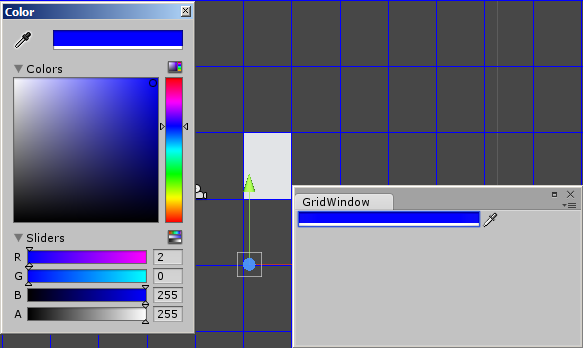As you can see, we'll be creating an editor window, and a color picker whose selection we'll use to draw a grid. We'll also be able to create and delete objects, snapped to this grid, and undo such actions.

First we'll learn how to use gizmos. Here are a few examples of built-in gizmos.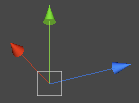This is the one you'll probably see the most in Unity, since it's drawn for every object that has a `Transform` component attached to it - so basically every selected object will have this gizmo drawn.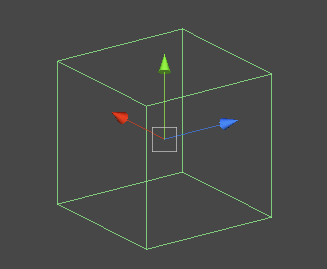Here's another gizmo, which enables us to see the size of the `BoxCollider` attached to our game object.

Create a C# script that we can use to draw our own gizmo for an object; we'll draw a simple grid in the editor as an example.

 01 02 03 04 05 06 07 08 09 10 11 12 13 14 `using` ``` UnityEngine;``` `using` ``` System.Collections;```   `public` ``` class``` ``` Grid : MonoBehaviour ``` `{` `    `  `    ``void` ``` Start () ``` `    ``{` `    ``}` `    `  `    ``void` ``` Update () ``` `    ``{` `    ``}` `}`

For a grid we need to add two variables, the width and the height.

 01 02 03 04 05 06 07 08 09 10 11 12 13 `public` ``` class``` ``` Grid : MonoBehaviour ``` `{` `    ``public` ``` float``` ``` width = 32.0f;``` `    ``public` ``` float``` ``` height = 32.0f;``` `    `  `    ``void` ``` Start () ``` `    ``{` `    ``}` `    `  `    ``void` ``` Update () ``` `    ``{` `    ``}` `}`

To draw in the editor we need to use `OnDrawGizmos` callback, so let's create it.

 01 02 03 04 05 06 07 08 09 10 11 12 13 14 15 16 17 `public` ``` class``` ``` Grid : MonoBehaviour ``` `{` `    ``public` ``` float``` ``` width = 32.0f;``` `    ``public` ``` float``` ``` height = 32.0f;``` `    `  `    ``void` ``` Start () ``` `    ``{` `    ``}` `    `  `    ``void` ``` Update () ``` `    ``{` `    ``}` `    `  `    ``void` ``` OnDrawGizmos()``` `    ``{` `    ``}` `}`

To draw a grid we need a set of horizontal and vertical lines and the position of the editor's camera so we know around which point we should draw our grid. First, let's save the camera's position to a separate variable.

 1 2 3 4 `void` ``` OnDrawGizmos()``` `{` `    ````Vector3 pos = Camera.current.transform.position;``` `}`

As you can see, we can get the editor's camera by using the `Camera.current` reference.

Now we'll need two for loops that will draw the horizontal and vertical lines.

 01 02 03 04 05 06 07 08 09 10 11 12 13 14 15 16 `void` ``` OnDrawGizmos()``` `{` `    ````Vector3 pos = Camera.current.transform.position;``` `    `  `    ``for` ``` (````float` ``` y = pos.y - 800.0f; y < pos.y + 800.0f; y+= height)``` `    ``{` `        ``Gizmos.DrawLine(``new` ``` Vector3(-1000000.0f, Mathf.Floor(y/height) * height, 0.0f),``` `                        ``new` ``` Vector3(1000000.0f, Mathf.Floor(y/height) * height, 0.0f));``` `    ``}` `   `  `    ``for` ``` (````float` ``` x = pos.x - 1200.0f; x < pos.x + 1200.0f; x+= width)``` `    ``{` `        ``Gizmos.DrawLine(``new` ``` Vector3(Mathf.Floor(x/width) * width, -1000000.0f, 0.0f),``` `                        ``new` ``` Vector3(Mathf.Floor(x/width) * width, 1000000.0f, 0.0f));``` `    ``}` `}`

To draw lines we use `Gizmos.DrawLine()`. Note that the `Gizmos` class has a lot of other drawing API methods, so it is possible to draw such primitives as cube or sphere or even their wireframes. You can also draw an image if you need to.

The grid lines should be infinitely long but `float.positiveInfinity` and`float.negativeInfinity` didn't seem to work well with drawing the lines, so we can simply put arbitrarily large numbers instead of those. Also, the number of lines strictly depend on the constants we put in the `for` loops' definitions; technically we shouldn't leave those constants like that but it's just a test code.

To see the grid, create an empty object and attach our script to it: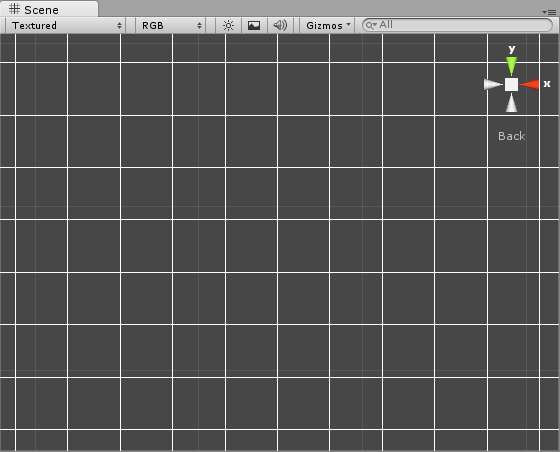The next thing to cover is customizing the inspector. To do that we need to create an editor script. Create a new C# file and name it GridEditor. This script should be placed in the Editor folder; if you don't have one then create it now.

 1 2 3 4 5 6 7 8 `using` ``` UnityEngine;``` `using` ``` UnityEditor;``` `using` ``` System.Collections;```   ```[CustomEditor (````typeof``(Grid))]` `public` ``` class``` ``` GridEditor : Editor``` `{` `}`

This time we also need to use `UnityEditor` to be able to make use of the editor classes and functions. To override the default inspector of our `Grid` object we need to add an attribute before our class declaration, `[CustomEditor (typeof(Grid))]` lets Unity know that we'll be customising the `Grid`'s inspector. To be able to use the editor callbacks, we need to derive from the `Editor` class instead of `MonoBehaviour`.

To change the current inspector we need to override the old one.

 1 2 3 4 5 6 `public` ``` class``` ``` GridEditor : Editor``` `{` `    ``public` ``` override``` ``` void``` ``` OnInspectorGUI()``` `    ``{` `    ``}` `}`

If you check the grid object's inspector in the editor now, it'll be empty even though the object itself has some public members. That's because by overriding the `OnInspectorGUI()`we discarded the default inspector in order to make a custom one instead.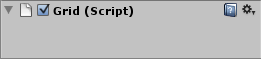Before we create any fields we need to get a reference to the object that inspector applies to. We actually have its reference already - it's named `target` - but for convenience we'll create a reference to the `Grid` component of that object. First, let's declare it.

 1 2 3 `public` ``` class``` ``` GridEditor : Editor``` `{` `    ````Grid grid;```

We should assign it in `OnEnable()` function which is called as soon as the inspector is enabled.

 1 2 3 4 5 6 7 8 `public` ``` class``` ``` GridEditor : Editor``` `{` `    ````Grid grid;```   `    ``public` ``` void``` ``` OnEnable()``` `    ``{` `        ````grid = (Grid)target;``` `    ``}`

Let's create some inspector fields now. We'll use GUILayout and EditorGUILayout classes for that.

 1 2 3 4 5 6 7 `public` ``` override``` ``` void``` ``` OnInspectorGUI()``` `{` `    ``GUILayout.BeginHorizontal();` `    ``GUILayout.Label(````" Grid Width "````);` `    ````grid.width = EditorGUILayout.FloatField(grid.width, GUILayout.Width(50));``` `    ``GUILayout.EndHorizontal();` `}`

The first line, `GUILayout.BeginHorizontal();` indicates that we want to place the following inspector elements next to each other, left to right. As you may imagine, the last line,`GUILayout.EndHorizontal();` indicates that we no longer want to do that. The actual items are in between those two lines. The first one is a simple label (in our case it will be displaying Grid Width text), and then next to it we create a `EditorGUILayout.FloatField`which is as you may imagine a float field. Note that we're assigning `grid.width` to the value of that `FloatField`, and the float field itself shows the value of `grid.width`. We also set its width to `50` pixels.

Let's see if the field is added to the inspector:Now let's add one more item to the inspector; this time it will be `grid.height`.

 01 02 03 04 05 06 07 08 09 10 11 12 `public` ``` override``` ``` void``` ``` OnInspectorGUI()``` `{` `    ``GUILayout.BeginHorizontal();` `    ``GUILayout.Label(````" Grid Width "````);` `    ````grid.width = EditorGUILayout.FloatField(grid.width, GUILayout.Width(50));``` `    ``GUILayout.EndHorizontal();` `    `  `    ``GUILayout.BeginHorizontal();` `    ``GUILayout.Label(````" Grid Height "````);` `    ````grid.height = EditorGUILayout.FloatField(grid.height, GUILayout.Width(50));``` `    ``GUILayout.EndHorizontal();` `}`

That'd be all for our grid object fields, if you want to know about other fields and items that you can use in the inspector then you can visit the Unity reference pages onEditorGUILayout and GUILayout.

Note that the changes we make in our new inspector are visible only after we select the Scene View window. To make them visible once they are made we may call`SceneView.RepaintAll()`.

 01 02 03 04 05 06 07 08 09 10 11 12 13 14 `public` ``` override``` ``` void``` ``` OnInspectorGUI()``` `{` `    ``GUILayout.BeginHorizontal();` `    ``GUILayout.Label(````" Grid Width "````);` `    ````grid.width = EditorGUILayout.FloatField(grid.width, GUILayout.Width(50));``` `    ``GUILayout.EndHorizontal();` `    `  `    ``GUILayout.BeginHorizontal();` `    ``GUILayout.Label(````" Grid Height "````);` `    ````grid.height = EditorGUILayout.FloatField(grid.height, GUILayout.Width(50));``` `    ``GUILayout.EndHorizontal();` `    `  `    ``SceneView.RepaintAll();` `}`

Now we don't have to click outside the inspector to see the results of the changes.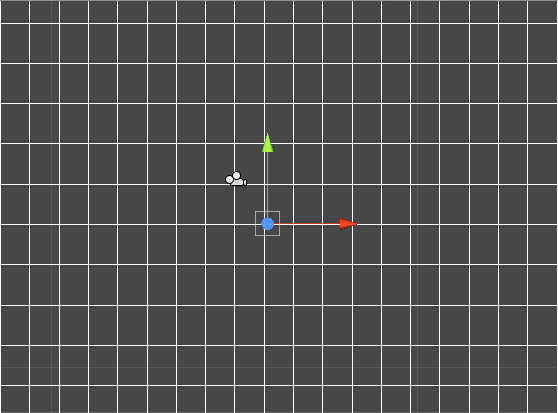Now let's try to handle the editor's input, just like we would do it in the game. Any key or mouse states should be available to us. To have this functionality we have to add a`onSceneGUIDelegate` callback to our `SceneView`. Let's call our update function `GridUpdate()`.

 1 2 3 4 5 6 7 8 9 `public` ``` void``` ``` OnEnable()``` `{` `    ````grid = (Grid)target;``` `    ````SceneView.onSceneGUIDelegate = GridUpdate;``` `}`   `void` ``` GridUpdate(SceneView sceneview)``` `{` `}`

Now we only need to get the input `Event`.

 1 2 3 4 `void` ``` GridUpdate(SceneView sceneview)``` `{` `    ````Event e = Event.current;``` `}`

For further playing with the editor scripts we'll need a game object that we'll be able to use. Let's create a simple cube and make a prefab out of it.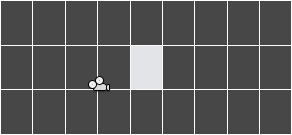You can match the size of the grid to the cube or the other way around and align it with a grid.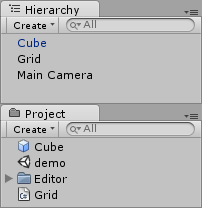As you can see, in the hierarchy view the cube text is colored in blue; this means it's connected to a prefab. You can see that prefab in the Project window.

Now we'll create an object from the editor script. Let's go back to our GridEditor.cs and extend the `GridUpdate()` function.

Let's create the object when the key a is pressed.

 1 2 3 4 5 6 7 8 9 `void` ``` GridUpdate(SceneView sceneview)``` `{` `    ````Event e = Event.current;``` `    `  `    ``if` ``` (e.isKey && e.character == ````'a'``)` `    ``{` `        ````GameObject obj;``` `    ``}` `}`

As you can see, we simply check whether the event is a key state change and whether the character that was pressed is '`a`'. We also create a reference for our new object. Now let's instantiate it.

 01 02 03 04 05 06 07 08 09 10 11 12 13 14 `void` ``` GridUpdate(SceneView sceneview)``` `{` `    ````Event e = Event.current;``` `    `  `    ``if` ``` (e.isKey && e.character == ````'a'``)` `    ``{` `        ````GameObject obj;``` `        ``if` ``` (Selection.activeObject)``` `        ``{` `            ````obj = (GameObject)Instantiate(Selection.activeObject);``` `            ````obj.transform.position = ````new` ``` Vector3(0.0f, 0.0f, 0.0f);``` `        ``}` `    ``}` `}`

`Selection.activeObject` is a reference to the currently selected object in the editor. If any object is selected then we simply clone it and change the clone's position to ```(0.0, 0.0, 0.0)```.

Let's test whether it works. You must be mindful of one thing: our `GridUpdate()` stops working whenever the assets are reimported/refreshed, and to re-enable it you have to select the object (for example from the hierarchy view) that the editor script refers to - in our example it's the Grid object. You also need to remember that the input events will be caught only if the Scene view is selected.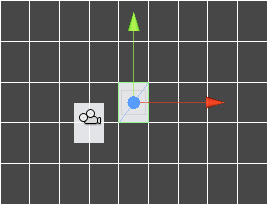Altough we managed to clone the object, the cloned object's link to the prefab is non existant.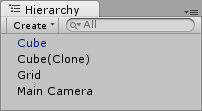As you can see, the Cube (Clone) name is displayed with plain black font and that means it's not connected to the prefab as the original cube is. If we were to duplicate the original cube manually in the editor, the cloned cube would be linked to the Cubeprefab. To make it work this way for us we need to use `InstantiatePrefab()` function from`EditorUtility` class.

Before we use this function we need to get the selected object's prefab. To do that we need to use `GetPrefabParent()` which also belongs to the `EditorUtility` class.

 01 02 03 04 05 06 07 08 09 10 11 `void` ``` GridUpdate(SceneView sceneview)``` `{` `    ````Event e = Event.current;``` `    `  `    ``if` ``` (e.isKey&& e.character == ````'a'``)` `    ``{` `        ````GameObject obj;``` `        ````Object prefab = EditorUtility.GetPrefabParent(Selection.activeObject);``` `        `  `        ``if` ``` (prefab)``` `        ``{`

We can also stop checking whether the `Selection.activeObject` exists, because if it doesn't then the `prefab` will be equal to `null`, and therefore we can get away with checking only the `prefab` reference.

Now let's instantiate our prefab and set its position.

 01 02 03 04 05 06 07 08 09 10 11 12 13 14 15 16 `void` ``` GridUpdate(SceneView sceneview)``` `{` `    ````Event e = Event.current;```   `    ``if` ``` (e.isKey && e.character == ````'a'``)` `    ``{` `        ````GameObject obj;``` `        ````Object prefab = EditorUtility.GetPrefabParent(Selection.activeObject);``` `        `  `        ``if` ``` (prefab)``` `        ``{` `            ````obj = (GameObject)EditorUtility.InstantiatePrefab(prefab);``` `            ````obj.transform.position = ````new` ``` Vector3(0.0f, 0.0f, 0.0f);``` `        ``}` `    ``}` `}`

And that's it - let's check whether the cloned cube is linked to the prefab now.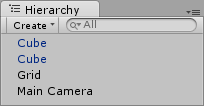The `Event` class doesn't let us know where the mouse is in the world space, it only provides the screen space mouse coordinates. Here's how we convert them so we can get an approximated world space mouse position.

 1 2 3 4 5 6 `void` ``` GridUpdate(SceneView sceneview)``` `{` `    ````Event e = Event.current;```   `    ````Ray r = Camera.current.ScreenPointToRay(````new` ``` Vector3(e.mousePosition.x, -e.mousePosition.y + Camera.current.pixelHeight));``` `    ````Vector3 mousePos = r.origin;```

First we use the editor's camera `ScreenPointToRay` to get the ray from the screen coordinates, but unfortunately before that we need to translate the event's screen space to a space that is acceptable for `ScreenPointToRay()`.

`e.mousePosition` holds the mouse position in a coordinate space where the top left corner is the `(0, 0)` point and bottom right corner is equal to ```(Camera.current.pixelWidth, -Camera.current.pixelHeight)```. We need to translate it into the space where the bottom left corner is the ```(0, 0)``` and the top right is `(Camera.current.pixelWidth, Camera.current.pixelHeight)`, which is pretty simple.

The next thing we should do is save the ray's origin to our `mousePos` vector, so it's easily accessible.

Now we can assign the clone's position to where the mouse is.

 1 2 3 4 5 `if` ``` (prefab)``` `{` `    ````obj = (GameObject)EditorUtility.InstantiatePrefab(prefab);``` `    ````obj.transform.position = ````new` ``` Vector3(mousePos.x, mousePos.y, 0.0f);``` `}`

Note that when the camera is set really flat then the approximation of mouse position on one of the axes is really really bad, that's why I set the `z` position of the clone manually. Now the cubes should be created wherever the mouse is.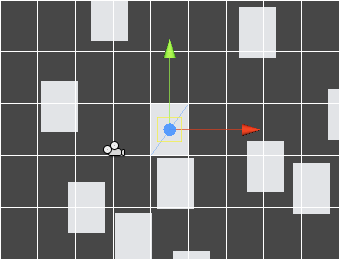Since we've got our grid set up, it would be a shame not to use it; let's use our mouse position to align the created cubes to the grid.

 1 2 3 4 5 6 7 `if` ``` (prefab)``` `{` `    ````obj = (GameObject)EditorUtility.InstantiatePrefab(prefab);``` `    ````Vector3 aligned = ````new` ``` Vector3(Mathf.Floor(mousePos.x/grid.width)*grid.width + grid.width/2.0f,``` `                                  ````Mathf.Floor(mousePos.y/grid.height)*grid.height + grid.height/2.0f, 0.0f);``` `    ````obj.transform.position = aligned;``` `}`

Take a look at the result: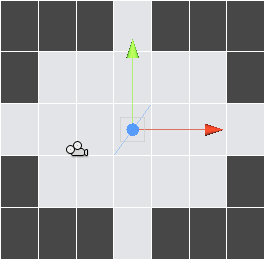In this step we'll delete objects programmatically in the editor. We can do that by using`DestroyImmediate()`. In this example let's make a greater use of the `Selection` class and delete all of the selected objects when the '`d`' key is pressed.

 01 02 03 04 05 06 07 08 09 10 11 12 13 14 15 16 17 18 `if` ``` (e.isKey && e.character == ````'a'``)` `{` `    ````GameObject obj;``` `    ````Object prefab = EditorUtility.GetPrefabParent(Selection.activeObject);``` `    `  `    ``if` ``` (prefab)``` `    ``{` `        ````obj = (GameObject)EditorUtility.InstantiatePrefab(prefab);``` `        ````Vector3 aligned = ````new` ``` Vector3(Mathf.Floor(mousePos.x/grid.width)*grid.width + grid.width/2.0f,``` `                                      ````Mathf.Floor(mousePos.y/grid.height)*grid.height + grid.height/2.0f, 0.0f);``` `        ````obj.transform.position = aligned;``` `    ``}` `}` `else` ``` if``` ``` (e.isKey && e.character == ````'d'``)` `{` `    ``foreach` ``` (GameObject obj ````in` ``` Selection.gameObjects)``` `        ``DestroyImmediate(obj);` `}`

When the '`d`' key is pressed we run through all the selected objects and delete each one of them. Of course we could also press Delete key in the editor to delete those objects, but then these wouldn't be deleted by our script. Test it in the editor.

In this step we'll make use of the `Undo` class, which basically lets us undo each action that our editor script does. Let's start by undoing the object creation.

To be able to destroy an object that we created in editor we need to call`Undo.RegisterCreatedObjectUndo()`. It takes two arguments: the first is the object that has been created and the second is the name of the undo. The name of the action that is going to be undone is always displayed under Edit->Undo `name`.

 1 2 3 4 5 6 7 8 `if` ``` (prefab)``` `{` `    ````obj = (GameObject)EditorUtility.InstantiatePrefab(prefab);``` `    ````Vector3 aligned = ````new` ``` Vector3(Mathf.Floor(mousePos.x/grid.width)*grid.width + grid.width/2.0f,``` `                                  ````Mathf.Floor(mousePos.y/grid.height)*grid.height + grid.height/2.0f, 0.0f);``` `    ````obj.transform.position = aligned;``` `    ````Undo.RegisterCreatedObjectUndo(obj, ``````"Create "``` ``` + obj.name); ``` `}`

If you create a few cubes using the a key and then try to undo now you'll notice that all the created cubes has been deleted. That's because all these created cubes went into a single undo event.

If we want to place every created object on another undo event and make it possible to undo creating them one by one we need to use `Undo.IncrementCurrentEventIndex()`.

 1 2 3 4 5 6 7 8 9 `if` ``` (prefab)``` `{` `    ``Undo.IncrementCurrentEventIndex();` `    ````obj = (GameObject)EditorUtility.InstantiatePrefab(prefab);``` `    ````Vector3 aligned = ````new` ``` Vector3(Mathf.Floor(mousePos.x/grid.width)*grid.width + grid.width/2.0f,``` `                                  ````Mathf.Floor(mousePos.y/grid.height)*grid.height + grid.height/2.0f, 0.0f);``` `    ````obj.transform.position = aligned;``` `    ````Undo.RegisterCreatedObjectUndo(obj, ``````"Create "``` ``` + obj.name); ``` `}`

If you test the script now you'll see that the cubes are deleted one by one by undoing their creation.

To undo the object deletion we have to use `Undo.RegisterSceneUndo()`. It's a very slow function that essentially saves the scene state so we can later revert to that state by performing an undo action. Unfortunately, it seems to be the only way for now to get the deleted objects back on the scene.

 1 2 3 4 5 6 7 `else` ``` if``` ``` (e.isKey && e.character == ````'d'``)` `{` `    ``Undo.IncrementCurrentEventIndex();` `    ``Undo.RegisterSceneUndo(````"Delete Selected Objects"````);` `    ``foreach` ``` (GameObject obj ````in` ``` Selection.gameObjects)``` `        ``DestroyImmediate(obj);` `}`

`Undo.RegisterSceneUndo()` takes only one argument, and that's the undo's name. After deleting a couple of cubes using the d key you can undo that deletion.

Create a new script, and let's make this one extend `EditorWindow` instead of `Editor`. Let's name it GridWindow.cs.

 01 02 03 04 05 06 07 08 09 10 `using` ``` UnityEngine;``` `using` ``` UnityEditor;``` `using` ``` System.Collections;```   `public` ``` class``` ``` GridWindow : EditorWindow``` `{` `    ``public` ``` void``` ``` Init()``` `    ``{` `    ``}` `}`

Let's create a reference to our Grid object so we can access it from the window.

 1 2 3 4 5 6 7 8 9 `public` ``` class``` ``` GridWindow : EditorWindow``` `{` `    ````Grid grid;``` `    `  `    ``public` ``` void``` ``` Init()``` `    ``{` `        ````grid = (Grid)FindObjectOfType(````typeof``(Grid));` `    ``}` `}`

Now we need to create the window, we can do that from our GridEditor script.

In our `OnInspectorGUI()` let's add a button that will create the `GridWindow`.

 01 02 03 04 05 06 07 08 09 10 11 12 13 14 15 16 17 18 19 20 `public` ``` override``` ``` void``` ``` OnInspectorGUI()``` `{` `    ``GUILayout.BeginHorizontal();` `    ``GUILayout.Label(````" Grid Width "````);` `    ````grid.width = EditorGUILayout.FloatField(grid.width, GUILayout.Width(50));``` `    ``GUILayout.EndHorizontal();` `    `  `    ``GUILayout.BeginHorizontal();` `    ``GUILayout.Label(````" Grid Height "````);` `    ````grid.height = EditorGUILayout.FloatField(grid.height, GUILayout.Width(50));``` `    ``GUILayout.EndHorizontal();` `    `  `    ``if` ``` (GUILayout.Button(``````"Open Grid Window"``````, GUILayout.Width(255)))``` `    ````{   ``` `       ````GridWindow window = (GridWindow) EditorWindow.GetWindow(````typeof``(GridWindow));` `       ``window.Init();` `    ``}` `    `  `    ``SceneView.RepaintAll();` `}`

We use `GUILayout` to create a button, we also set the button's name and width. The`GUILayout.Button` returns `true` when the button is pressed, if that's the case then we open our `GridWindow`.

You can go back to the editor and press the button in our `Grid` object inspector.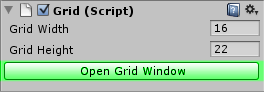Once you do that, the GridWindow should pop up.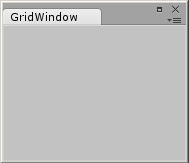Before we edit anything from our window, let's add a color field in our `Grid` class, so we can edit it later on.

 1 2 3 4 5 6 `public` ``` class``` ``` Grid : MonoBehaviour ``` `{` `    ``public` ``` float``` ``` width = 32.0f;``` `    ``public` ``` float``` ``` height = 32.0f;``` `    `  `    ``public` ``` Color color = Color.white;```

Now assign the `Gizmos.color` in the `OnDrawGizmos()` function.

 1 2 3 4 `void` ``` OnDrawGizmos()``` `{` `    ````Vector3 pos = Camera.current.transform.position;``` `    ````Gizmos.color = color;```

And now let's go back to GridWindow script and create a color field there so we can pick the color in the window. We can do that in the `OnGUI()` callback.

 01 02 03 04 05 06 07 08 09 10 11 12 13 14 `public` ``` class``` ``` GridWindow : EditorWindow``` `{` `    ````Grid grid;``` `    `  `    ``public` ``` void``` ``` Init()``` `    ``{` `        ````grid = (Grid)FindObjectOfType(````typeof``(Grid));` `    ``}` `    `  `    ``void` ``` OnGUI()``` `    ``{` `        ````grid.color = EditorGUILayout.ColorField(grid.color, GUILayout.Width(200));``` `    ``}` `}`

All right, now you can check whether everything works correctly in the editor:（注：此内容为转载，里面有些代码在新的unity已经废弃了，大家自己注意修改）

2d地图编辑器 unity3d 相关内容